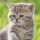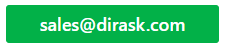Home
IT Knowledge
IT Career
Inspiration
Languages
EN

# Java date diff in minutes between 2 Date objects

1 pointsKate_C
19800

How can I calculate difference between two Date objects in minutes in java?

``````//                                   year  m  day  hour  min  sec nano
LocalDateTime of1 = LocalDateTime.of(2020, 1, 20,  16,   20,  0,  0);
LocalDateTime of2 = LocalDateTime.of(2020, 1, 20,  16,   35,  0,  0);

Date from = Date.from(of1.toInstant(ZoneOffset.UTC));
Date now  = Date.from(of2.toInstant(ZoneOffset.UTC));``````

The difference should be 15 minutes, as 35 - 20 = 15 minutes.
How to implement logic to get this diff?

3 pointsKate_C
19800

Quick solution:

``````public static long getDifferenceInMinutes(Date from, Date now) {
long diff = now.getTime() - from.getTime();
return diff / (60 * 1000);
}``````

Explanation based on question Date objects.
The difference is 15 min so:
15 min * 60 sec * 1000 ms = 900 000 ms
Each minute has 60 seconds.
Each seconds has 1000 milliseconds.

So the diff = 900 000 ms
In order to get time in minutes we need to divide it by
60 sec * 1000 milliseconds = 60 000 milliseconds - (60 * 1000) in our code.

900 000 / (60 * 1000) = 15 minutes

Also there should be easier way without explicit conversion with:
TimeUnit.MINUTES.convert, but it's nice to know what's under the hood.

## Full example

This example is based on the data from question. Both dates are the same and the difference is 15 min.

``````import java.time.LocalDateTime;
import java.time.ZoneOffset;
import java.util.Date;

public class DiffTimeExample {

public static void main(String[] args) {
//                                   year  m  day  hour  min  sec nano
LocalDateTime of1 = LocalDateTime.of(2020, 1, 20,  16,   20,  0,  0);
LocalDateTime of2 = LocalDateTime.of(2020, 1, 20,  16,   35,  0,  0);

Date from = Date.from(of1.toInstant(ZoneOffset.UTC));
Date now  = Date.from(of2.toInstant(ZoneOffset.UTC));

System.out.println(getDifferenceInMinutes(from, now)); // 15
// the difference between those 2 Date objects is 15 min

// Explanation:
// 15 min * 60 sec * 1000 ms = 900 000 ms
}

public static long getDifferenceInMinutes(Date from, Date now) {
long diff = now.getTime() - from.getTime();
return diff / (60 * 1000);
}
}``````

## Example 2

Second example shows that we can replace Date.from... with Date object. First Date from can be Date from database from entity, second can be current now.

``````import java.time.LocalDateTime;
import java.time.ZoneOffset;
import java.util.Date;

public class DiffTimeExample2 {

public static void main(String[] args) {
LocalDateTime of1 = LocalDateTime.of(2020, 1, 20,  16,   20,  0,  0);
Date from = Date.from(of1.toInstant(ZoneOffset.UTC)); // from db
Date now  = new Date(); // current time

System.out.println(getDifferenceInMinutes(from, now));
}

public static long getDifferenceInMinutes(Date from, Date now) {
long diff = now.getTime() - from.getTime();
return diff / (60 * 1000);
}
}``````